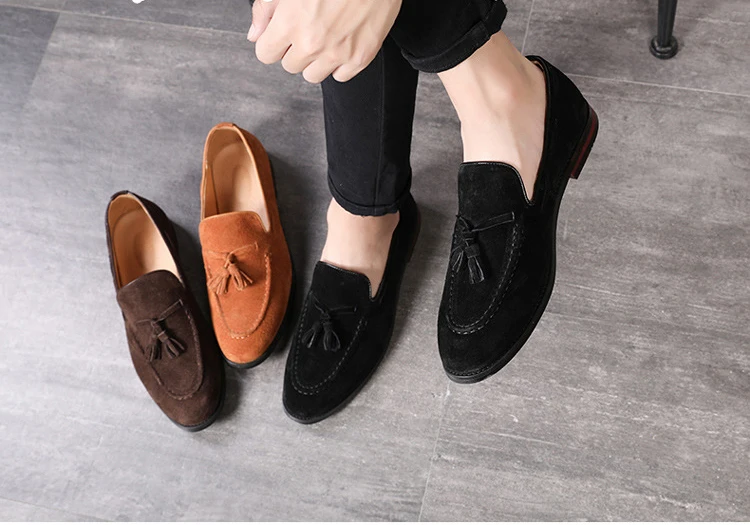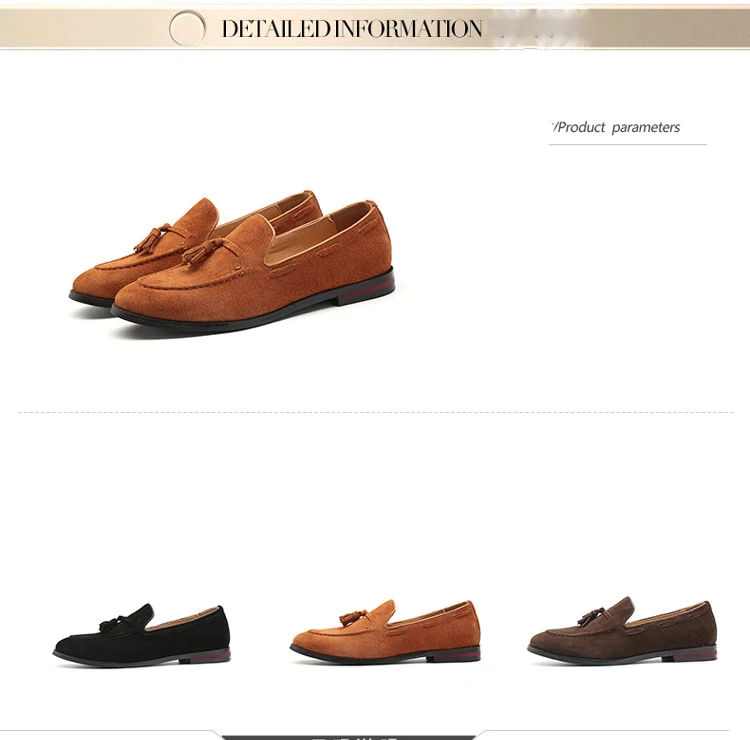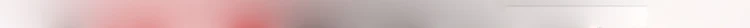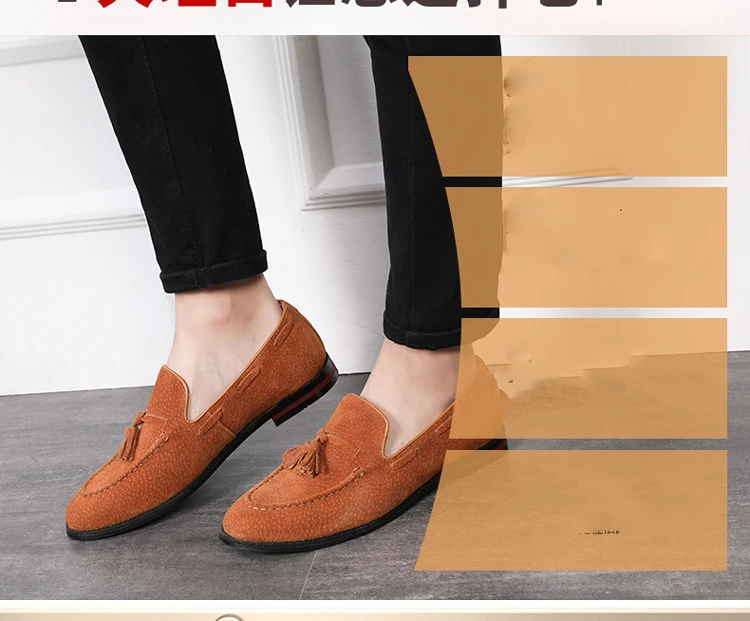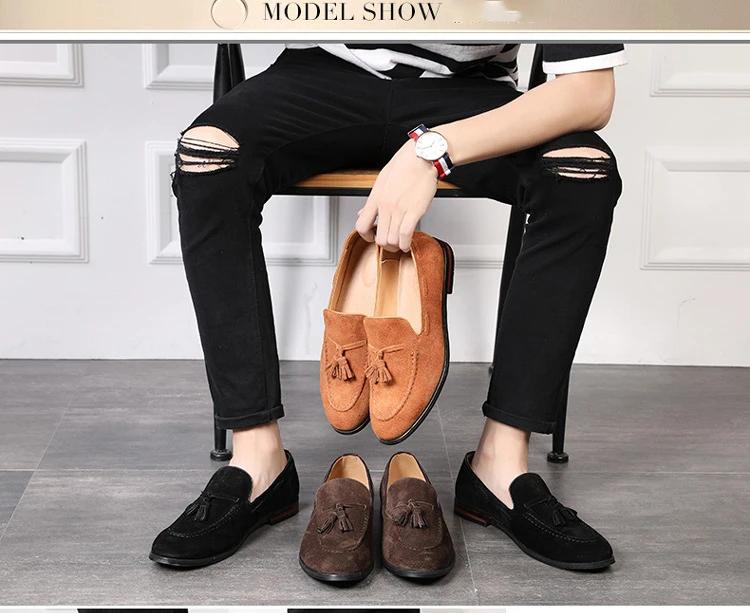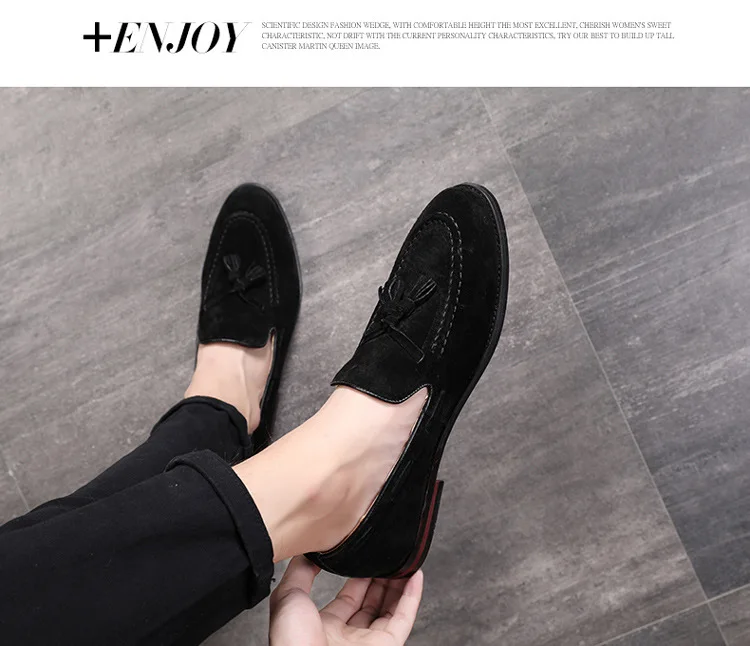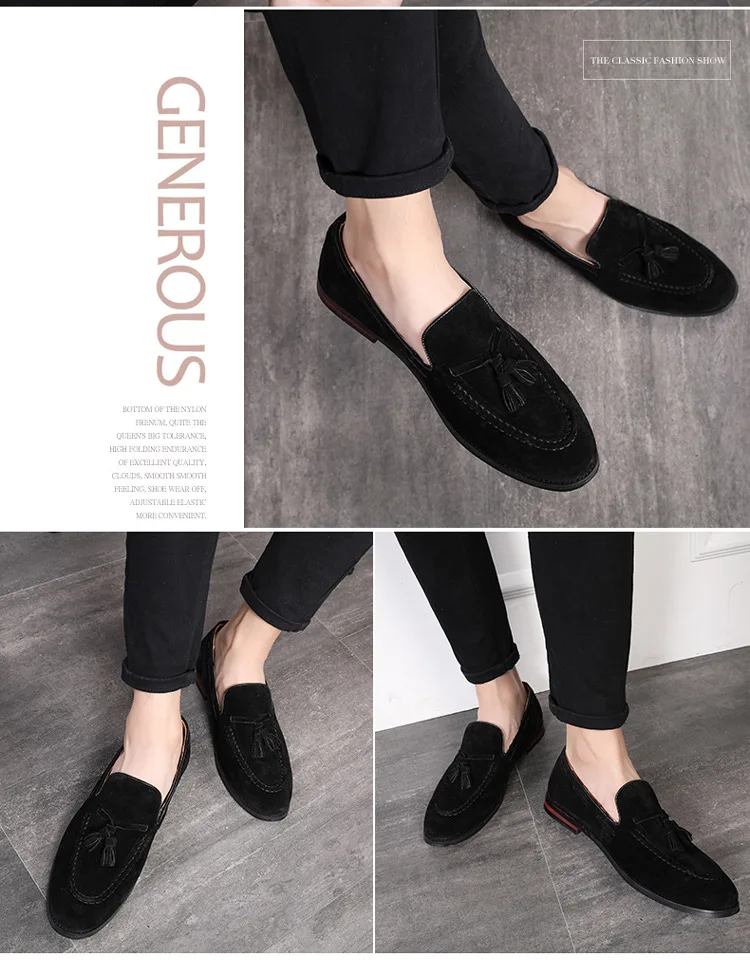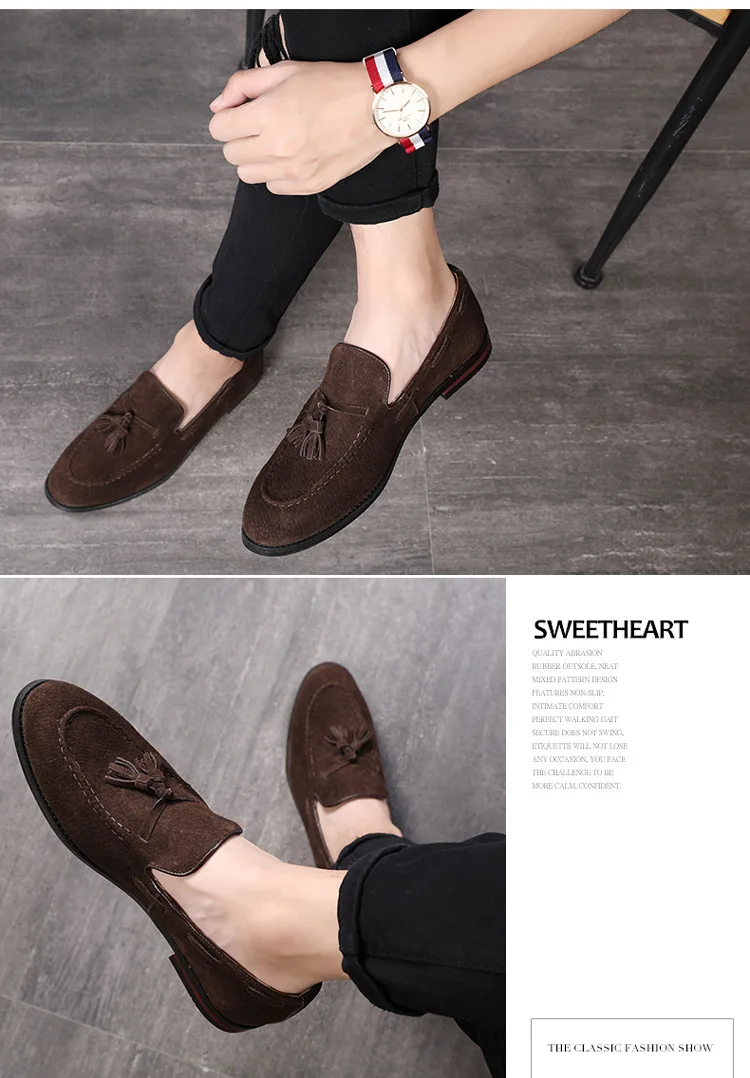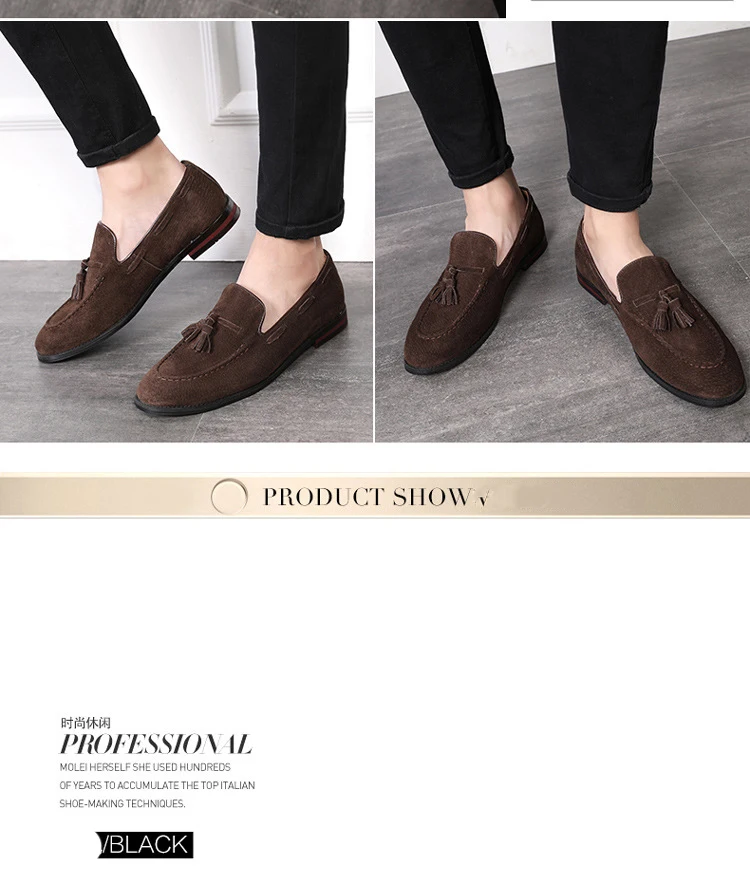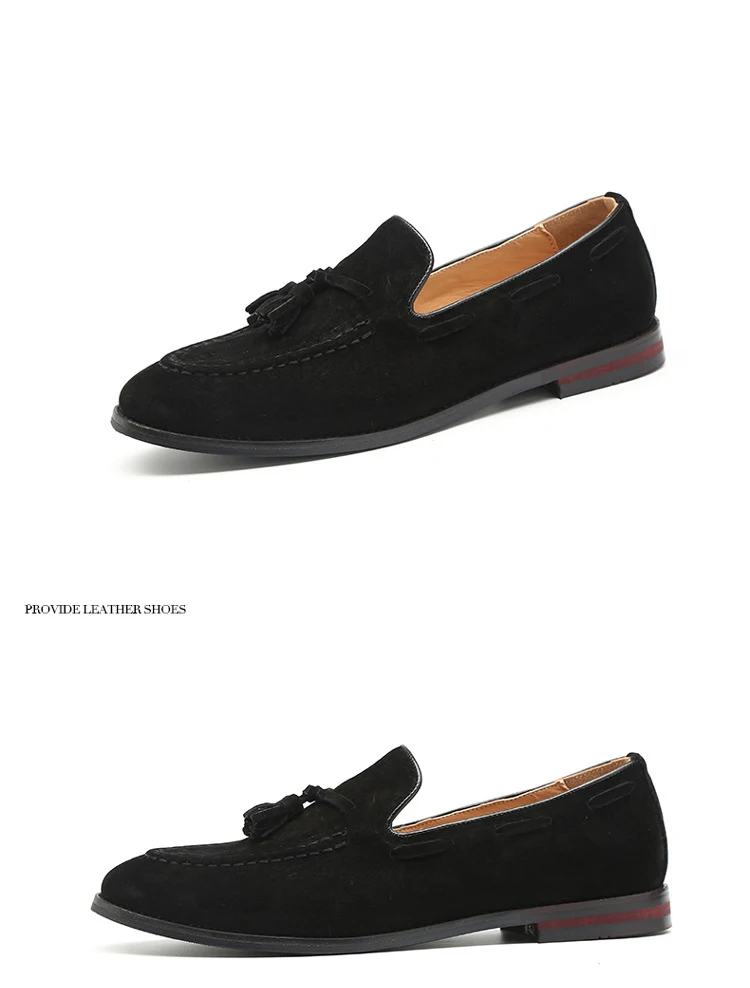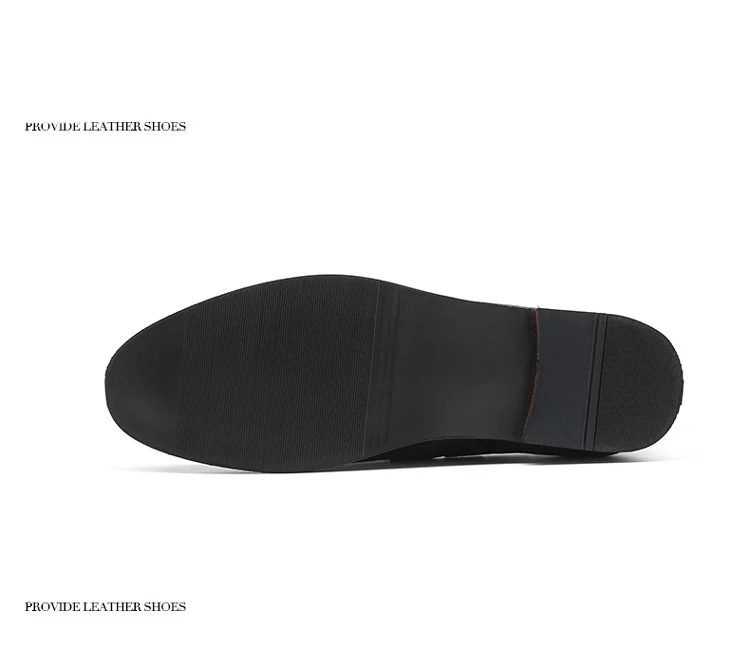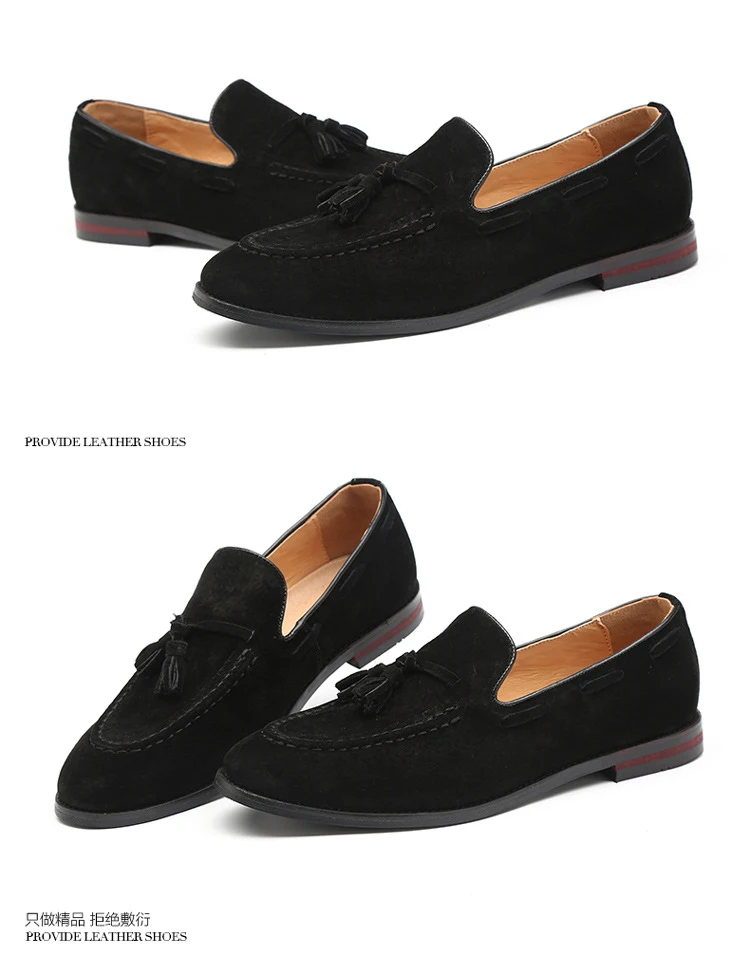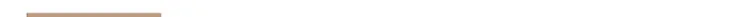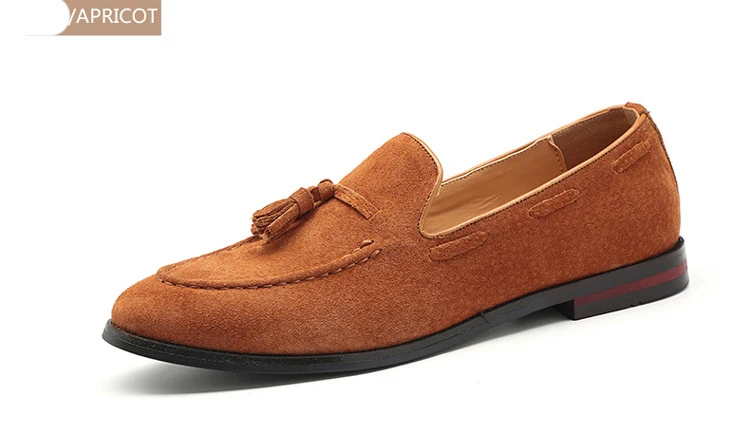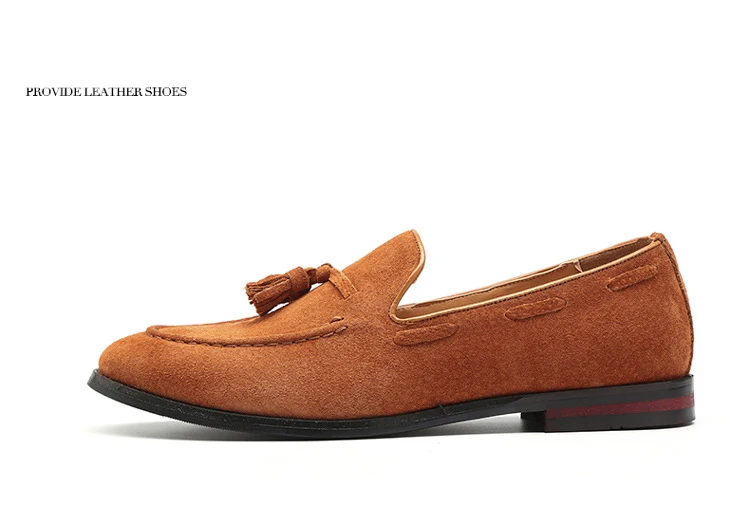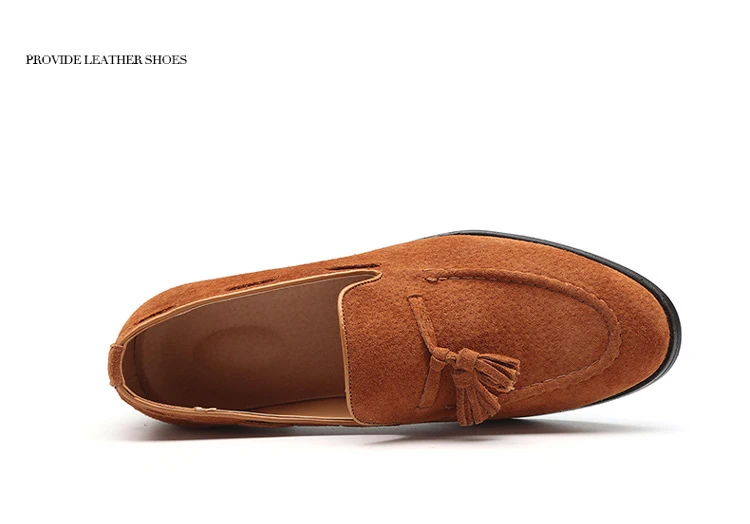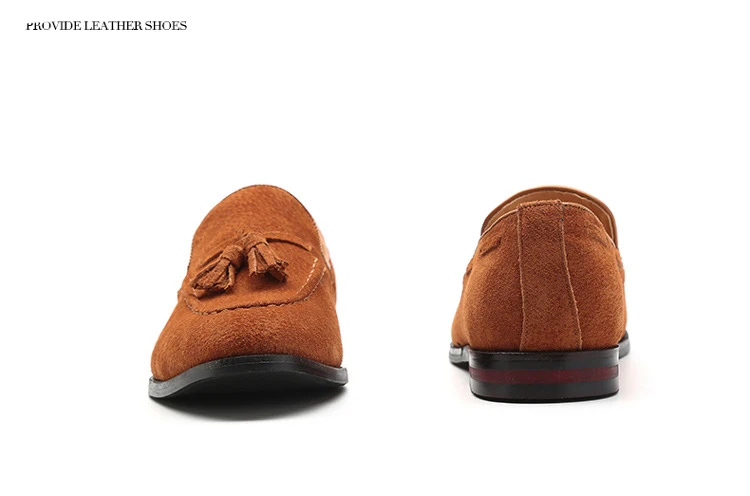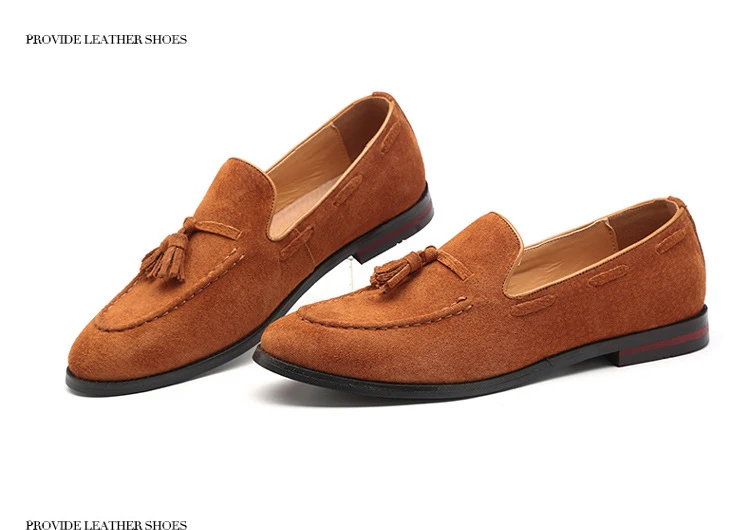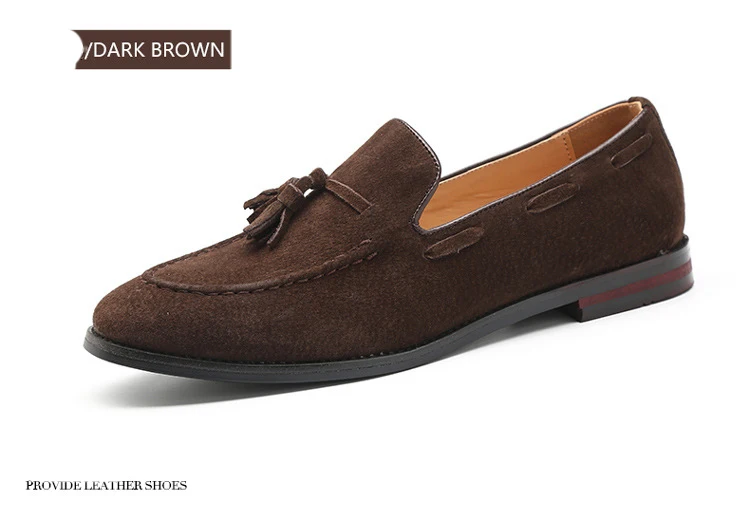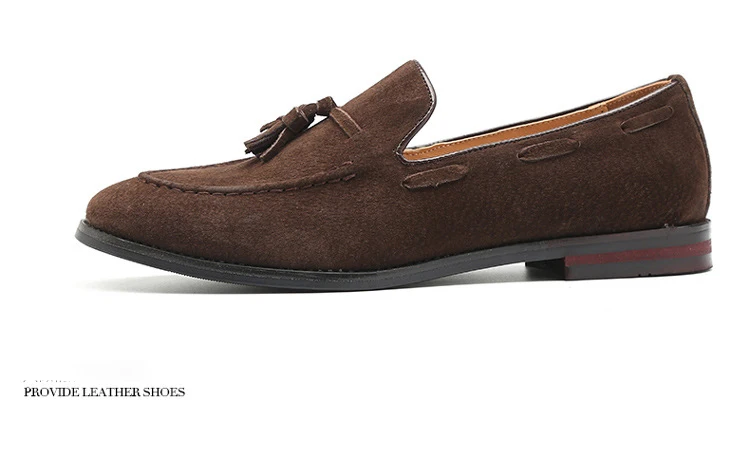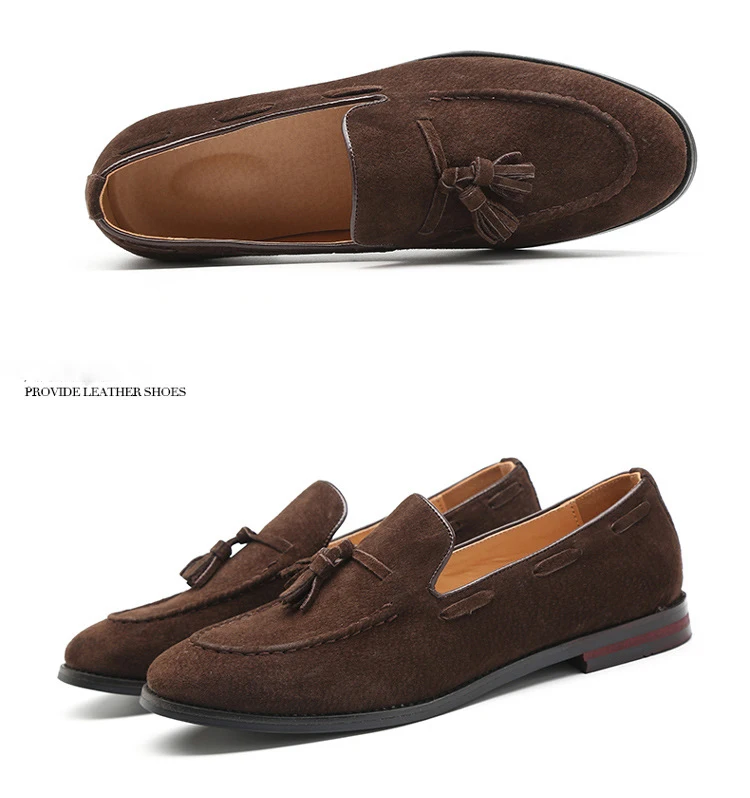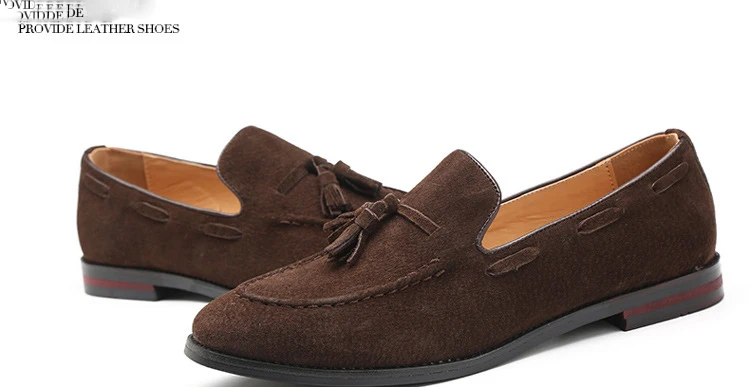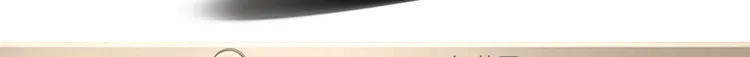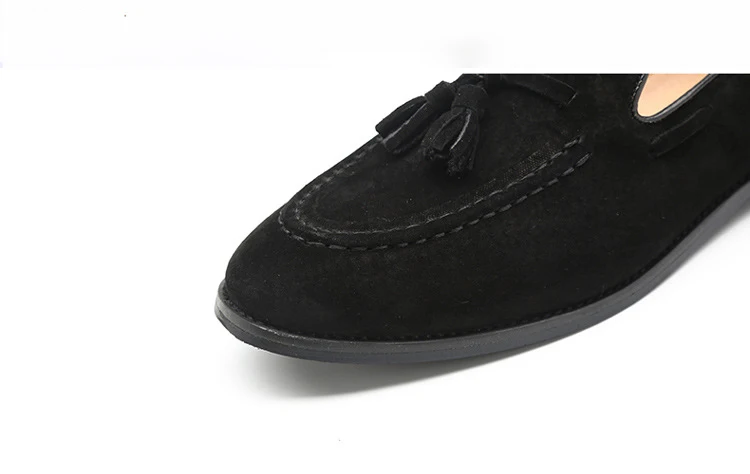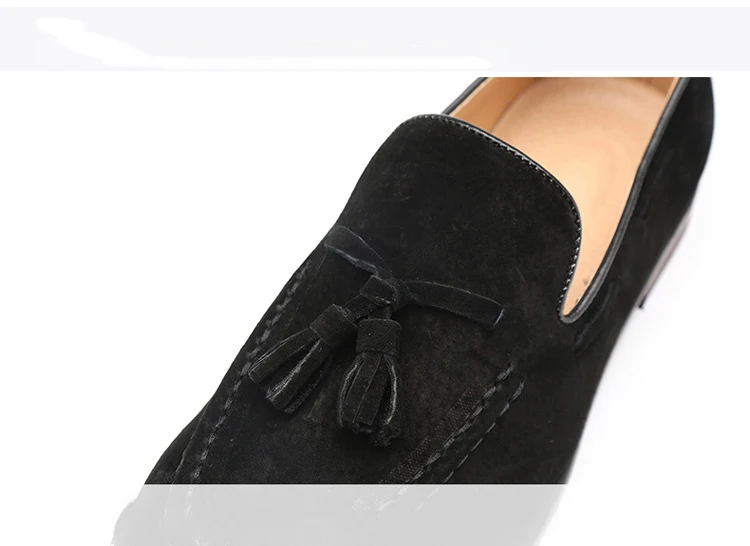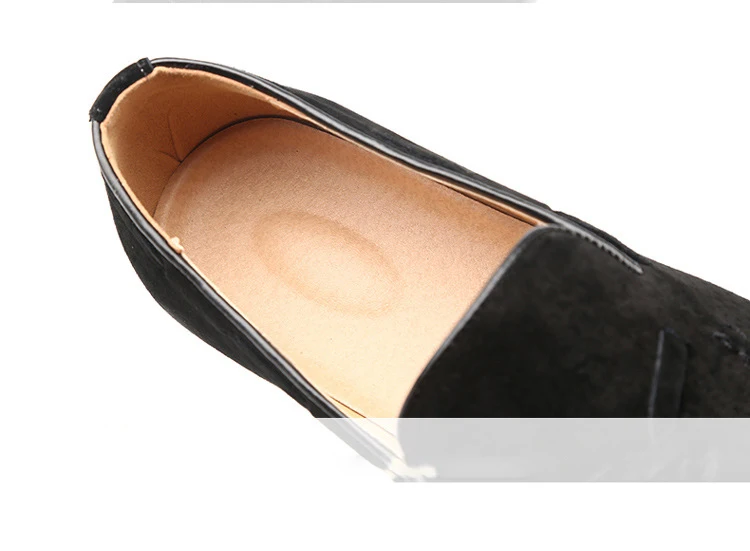Description

## Description

Big Size 37-48 Tassels Genuine Leather Casual Shoes Luxury Black Suede Men Loafers Moccasins Slippers Formal Wedding Dress Shoes

Size:4.5,5,5.5,6,6.5,7,8,8.5,9.5,10,11,12,12.5,13,35,36,37,38,39,40,41,42,43,44,45,46,47,48

1,Note Our size is not standard US size, and it is only equal to foot length!

US4.5 =EU35=225mm=8.85in(bare feet length)

US5 =EU36=230mm=9.05in

US5.5 =EU37=235mm=9.25in

US6.0 =EU38=240mm=9.45in

US6.5 =EU39=245mm=9.65in

US7.0 =EU40=250mm=9.84in

US8.0 =EU41=255mm=10.04in

US8.5 =EU42=260mm=10.24in

US9.5 =EU43=265mm=10.43in

US10.0=EU44=270mm=10.63in

US11.0=EU45=275mm=10.83in

US12.0=EU46=280mm=11.02in

US12.5=EU47=285mm=11.22in

US13.0=EU48=290mm=11.42in